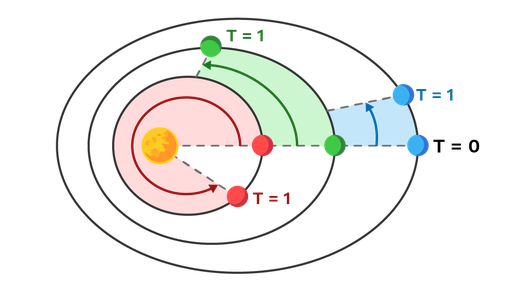Kepler’s third law relates the motion of different planets to one another. It is also called the law of harmonies. It states that:

The square of the orbital period of a planet (T) is directly proportional to the cube of the semimajor axis(a) of its orbit.

In equation form, it can be expressed as:

T2/a3=constant,

Or, alternatively, if we have two planets of orbital periods T and T’ and semimajor axes a and a’:

T2/a3= T’2/a’3.

Since this ratio is always the same, planets with bigger orbits (bigger a) will have longer years (bigger T): That is, it will take them longer to complete an orbit.

We call the constant of proportionality between T2 and a3 Kepler’s constant, or K. Its value is the same for the orbit of every planet in the Solar System. However, every orbital system will have its own value of K shared by every orbiting body in that system, but possibly different from that of other systems.Figure: By Kepler's third law, orbits with a bigger semimajor axis have longer periods. This means that, in the interval of time from T=0 to T=1, a planet with a smaller orbit would cover a bigger portion of its orbit.